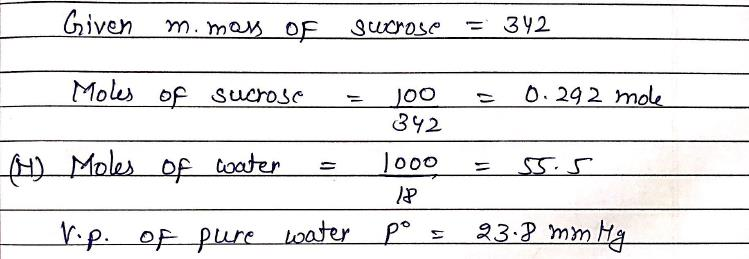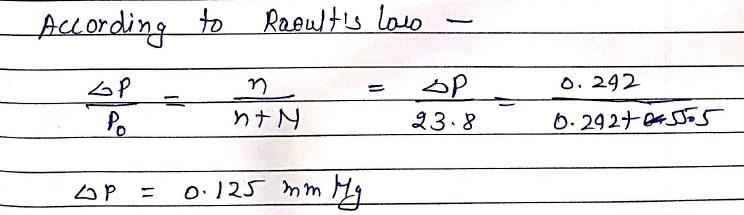Courses

# Mole - Volumetry - Redox - Colligative Properties

## 60 Questions MCQ Test MOCK Test Series for IIT JAM Chemistry | Mole - Volumetry - Redox - Colligative Properties

Description
This mock test of Mole - Volumetry - Redox - Colligative Properties for Chemistry helps you for every Chemistry entrance exam. This contains 60 Multiple Choice Questions for Chemistry Mole - Volumetry - Redox - Colligative Properties (mcq) to study with solutions a complete question bank. The solved questions answers in this Mole - Volumetry - Redox - Colligative Properties quiz give you a good mix of easy questions and tough questions. Chemistry students definitely take this Mole - Volumetry - Redox - Colligative Properties exercise for a better result in the exam. You can find other Mole - Volumetry - Redox - Colligative Properties extra questions, long questions & short questions for Chemistry on EduRev as well by searching above.
QUESTION: 1

### Consider a titration of Potassium dichromate solution with acidified Mohr’s salt solution using diphenylamine as indicator. The number of moles of Mohr’s salt required per mole of dichromate is:

Solution: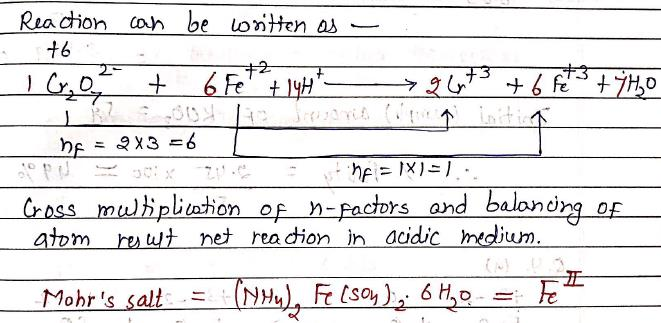QUESTION: 2

### Number of moles of NH3 formed when 0.535g of NH4Cl is completely decomposed by NaOH, is: NH4Cl + NaOH → NH3 + NaCl + H2O

Solution: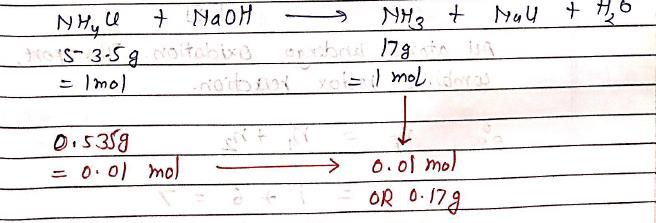QUESTION: 3

### 2KClO3 →2KCl + 3O2. 5g of KClO3 gave 0.03 mole of O2. The percentage purity of KClO3 is:

Solution: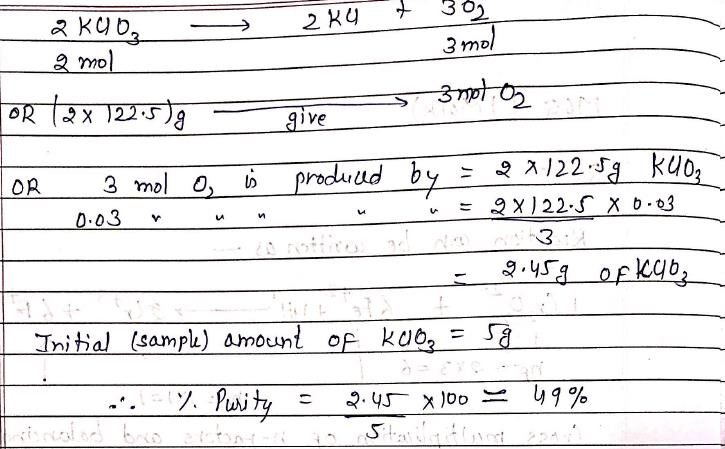QUESTION: 4

Calculate the equivalent Mass of FeS in the following reaction ?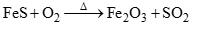Solution: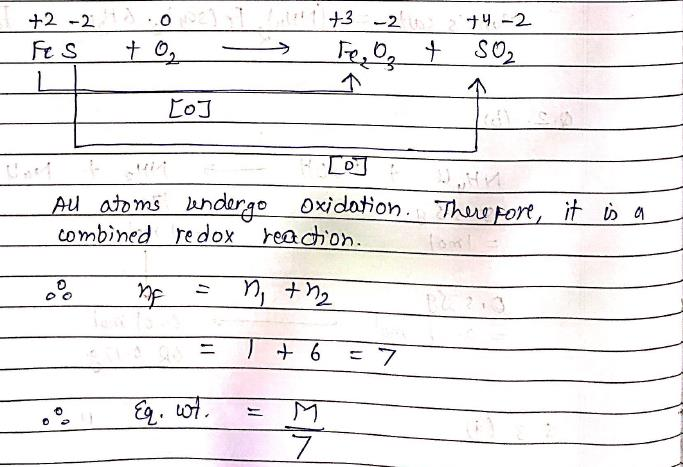QUESTION: 5

Which of the following acts as an oxidizing agent ?

Solution: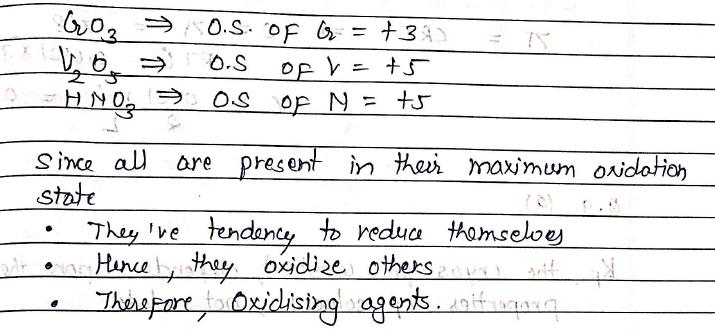QUESTION: 6

Vapor pressure of CHCl3 and CH2Cl2 at 250C are 200 mmHg and 41.5 mmHg resp. Vapor pressure of solution obtained by mixing 25.5g of CHCl3 and 40g of CH2Cl2 at same temp will be? (M.Wt. of CH2Cl2 = 85u and M.Wt. of CHCl3 = 119.5u)

Solution: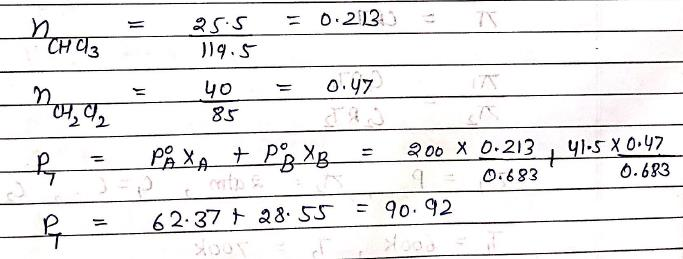QUESTION: 7

The relationship been Osmotic pressure at 273K when 10g Glucose (P1), 10g Urea (P2), 10g Sucrose (P3) are dissolved in 250 mL of water:

Solution: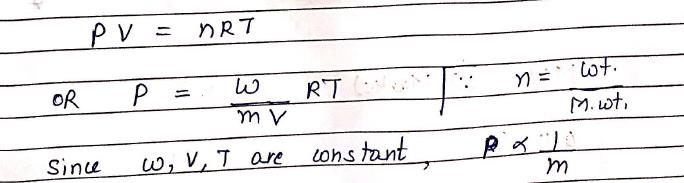QUESTION: 8

Average osmotic pressure of human blood is 7.8 bar at 370C. What is the concentration of an aqueous NaCl solution that could be used in blood stream? [R = 0.0821 JK-1mol-1]

Solution: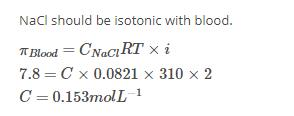QUESTION: 9

The value of Kf for water is 1.86, calculated from glucose solution. The value of Kf for water, calculated from NaCl solution will be (M.Wt of NaCl = 58.5 g/mol):

Solution:QUESTION: 10

At temp 3270C and concentration C, Osmotic pressure of a solution is P. The same solution at concentration C/2 and temp 4270C shows Osmotic pressure 2atm. Value of P will be:

Solution: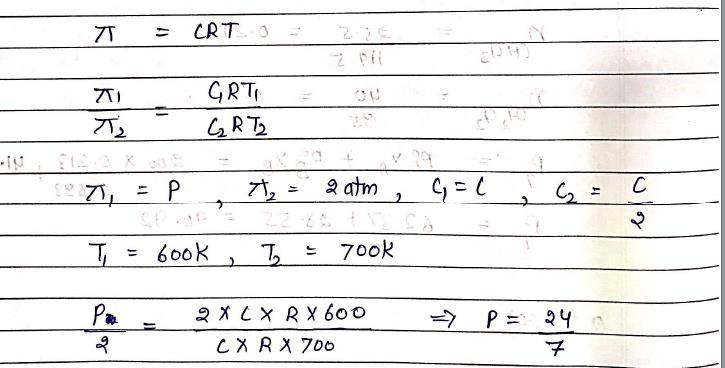QUESTION: 11

H2S2O8 and H2SO5 both have +6 oxidation state of sulfur. This is due to:

Solution: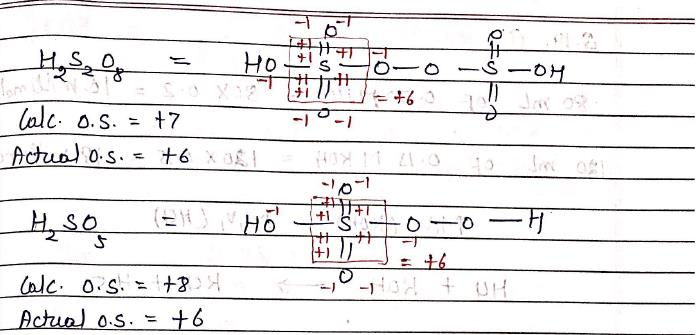QUESTION: 12

In an experiment, 20g of Vanadium oxide (molar mass 182 g/mol) was reduced by excess of Zinc dust in acidic solution to Vanadium (II) ions. The required number of moles of Iodine to re-oxidize Vanadium (II) to VO2+ is:

Solution: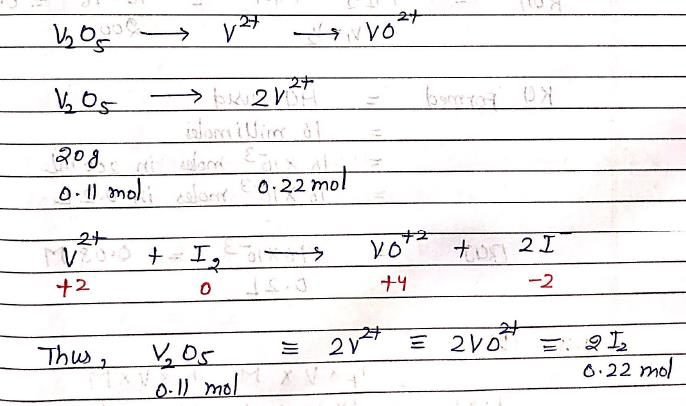QUESTION: 13

If CO2 is passed into waste water containing CrO4–2 (yellow), then, solution turns:

Solution: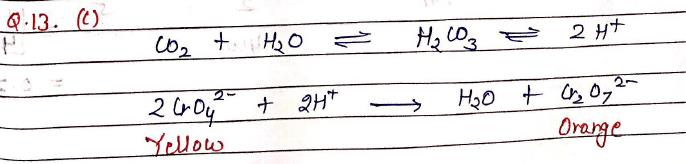QUESTION: 14

When 80 mL of 0.20 M HCl is mixed with 120 mL of 0.15 MKOH, the resulting solution is same as a solution of:

Solution: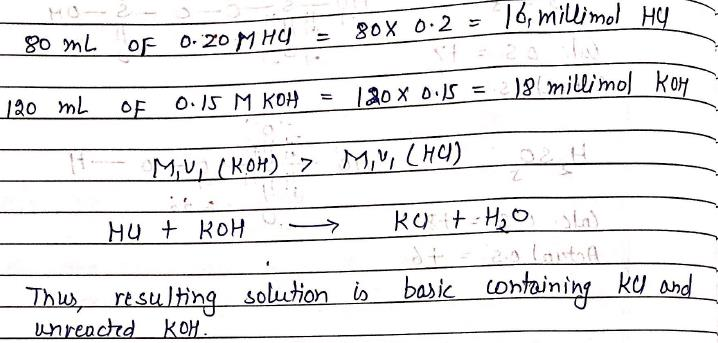QUESTION: 15

200 ml M/2 HNO3, 100 ml M/1 NaOH, 200 ml M/5 HCl are mixed together. The normality and the nature of mixture is:

Solution: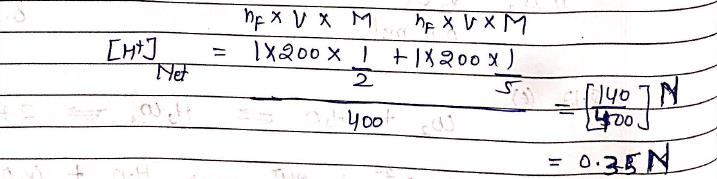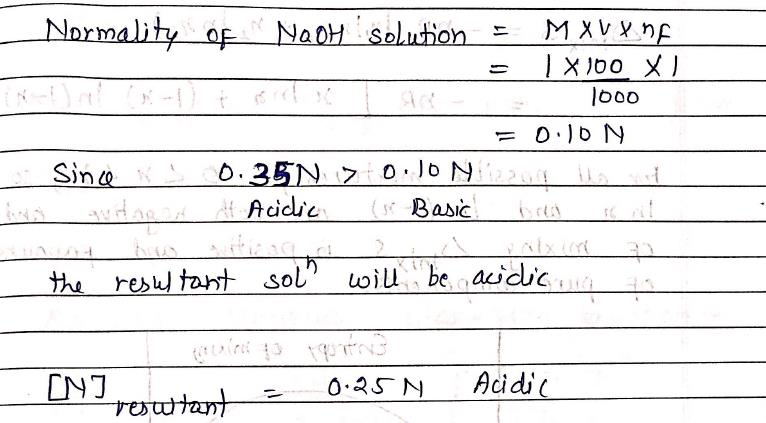QUESTION: 16

A 5.25% solution of a substance is isotonic with a 1.5 % solution of Urea in the same solvent. If density of both the solutions are assumed to be equal to 1.0g cm–3, molar mass of substance will be:

Solution: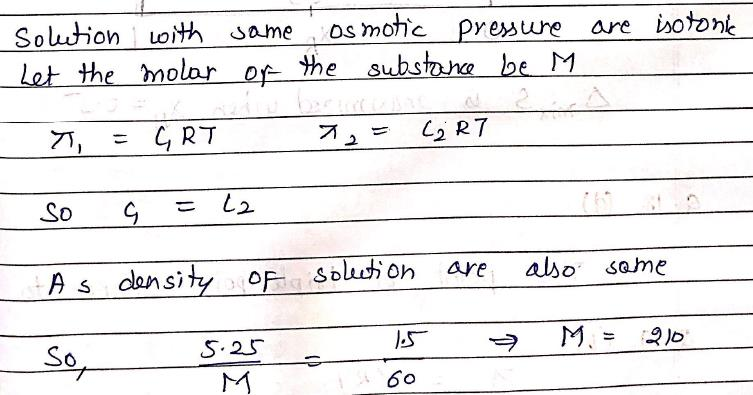QUESTION: 17

Which of the following condition is not satisfied by an ideal solution ?

Solution: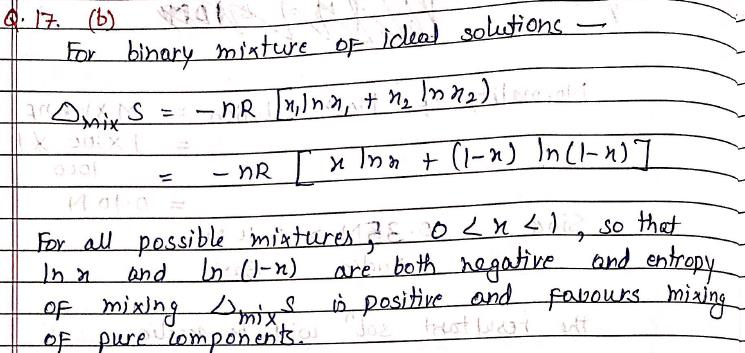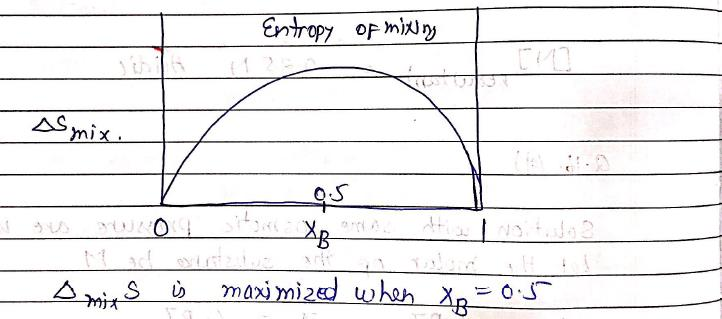QUESTION: 18

At a suitable pressure near the freezing point of water, there exists:

Solution: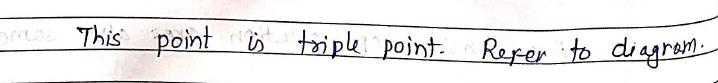QUESTION: 19

0.1 M NaCl and 0.05 M BaCl2 solutions are separated by a semi-permeable membrane in a container. For this system, choose the correct alternative:

Solution: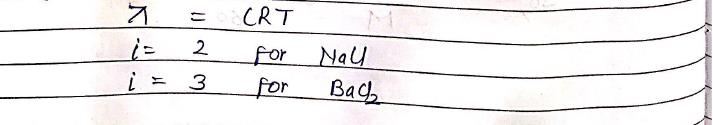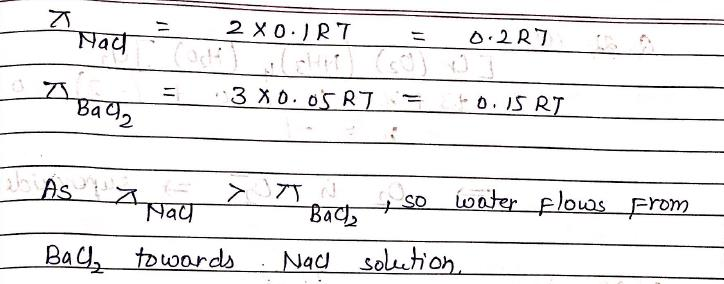QUESTION: 20

Normal boiling point of water is 373K (at 760mm). Vapor pressure of water at 298K is 23mm. If enthalpy of evaporation is 40656 J/mol, boiling point of water 23mm pressure will be:
[R = 8.314821 JK-1mol-1]

Solution: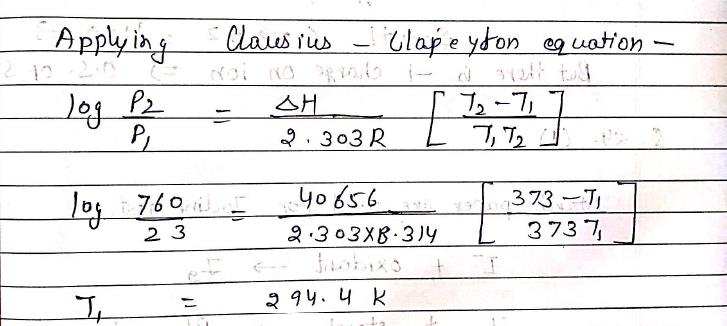QUESTION: 21

A 20 mL solution of Na2SO3 required 30 mL of 0.01 M Potassium dichromate solution for oxidation to Na2SO4. Hence, Molarity of Na2SO3 solution is:

Solution: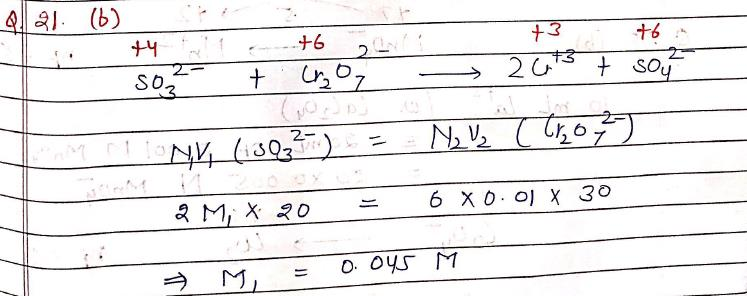QUESTION: 22

In [Cr(O2)(NH3)4(H2O)]Cl2, oxidation number of Cr is +3, the, O2 will be in form:

Solution: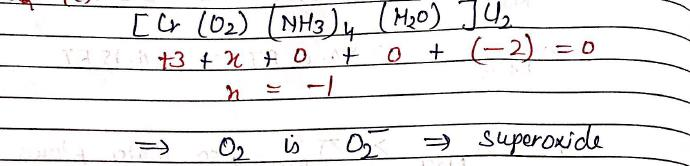QUESTION: 23

Oxidation state of sulfur in KSCN is (assume there is triple bond between C and N):

Solution: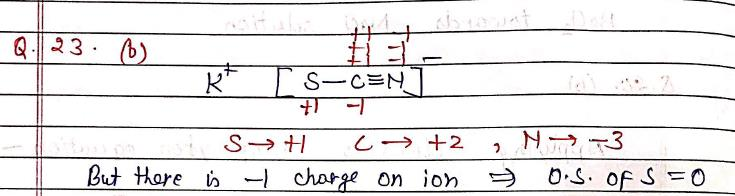QUESTION: 24

Starch paper is used to test the presence of:

Solution: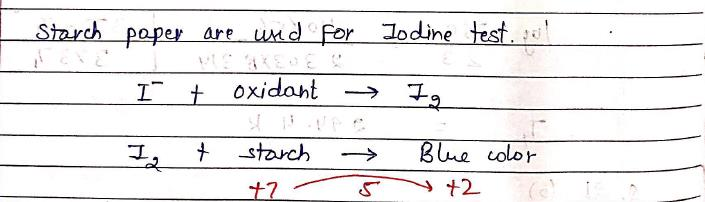QUESTION: 25

10 mL of a blood sample (containing Calcium oxalate) is dissolved in an acid. It required 20 mL of 0.001 M KMnO(which oxidizes oxalate to CO2). Hence, Ca2+ ion in 10 mL blood is:

Solution: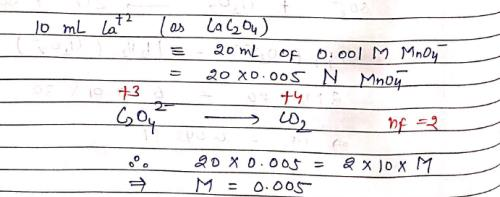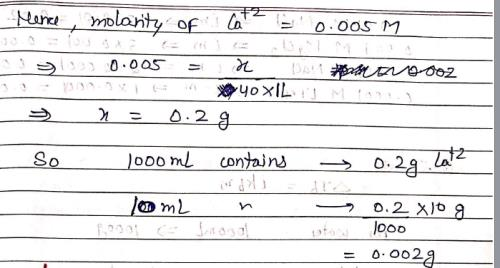QUESTION: 26

0.01 M solution of KCl and BaCl2 are prepared in water. The freezing point of KCl is found to be –20C. What is freezing point of BaCl2 solution when it is completely ionized?

Solution: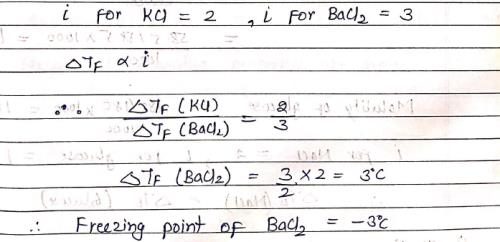QUESTION: 27

The correct order of increasing boiling points of the following aqueous solutions:
(I). 0.0001 M NaCl
(II). 0.0001 M Urea
(III). 0.001 MgCl2
(IV). 0.01 M NaCl

Solution: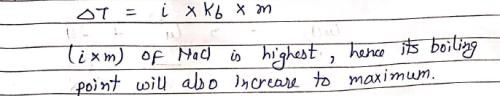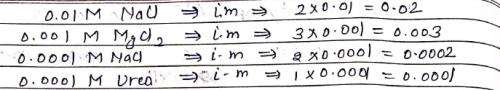QUESTION: 28

58.5 g of NaCl and 180 g of Glucose were separately dissolved in 1000 mL of water. Identify the correct statement regarding elevation of boiling point of resulting solutions:

Solution: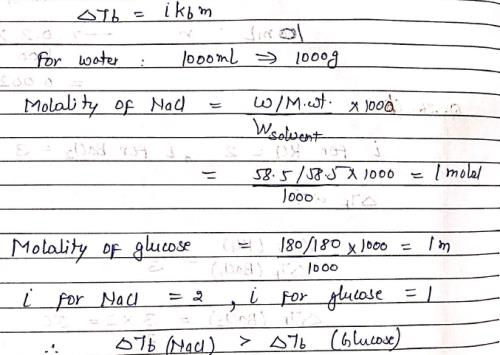QUESTION: 29

The elevation in boiling point of a solution of 13.44g of CuCl2 in 1Kg of water using following information will be ?
(M.Wt of CuCl2 = 134.4, Kb = 0.52 K molal–1, assume CuCl2 as a strong electrolyte):

Solution: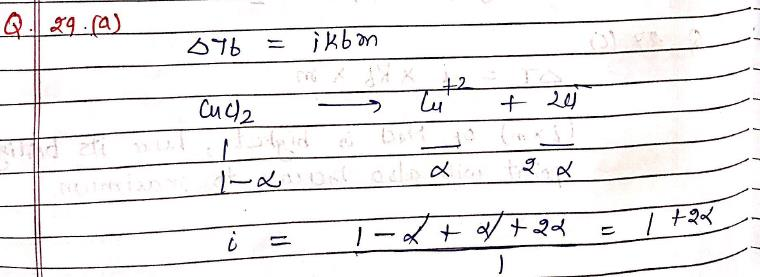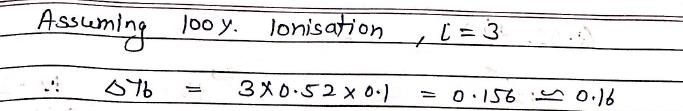QUESTION: 30

Consider separate solutions of 0.5M C2H5OH (aq), 0.1 M Mg3(PO4)2 (aq), 0.25 M KBr (aq), 0.125 M Na3PO4 (aq). Which statement is true about these solutions, assuming all salts to be strong electrolytes ?

Solution: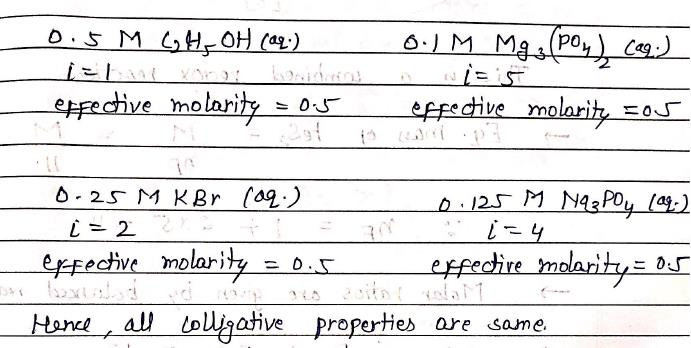*Multiple options can be correct
QUESTION: 31

We would expect no reaction in which of the following case(s)?

Solution: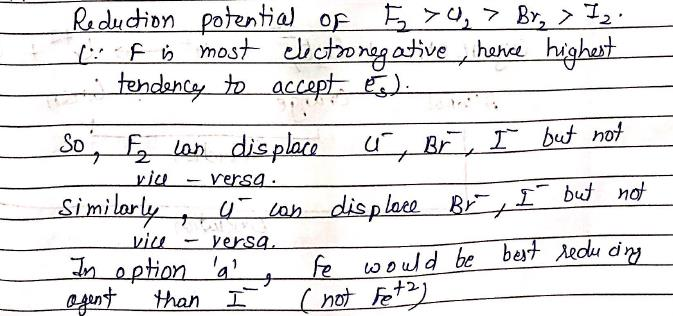*Multiple options can be correct
QUESTION: 32

In the reaction: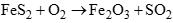Solution: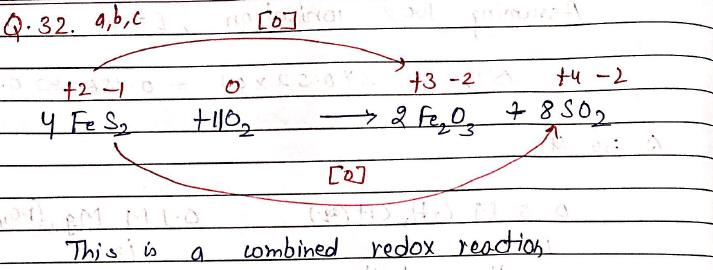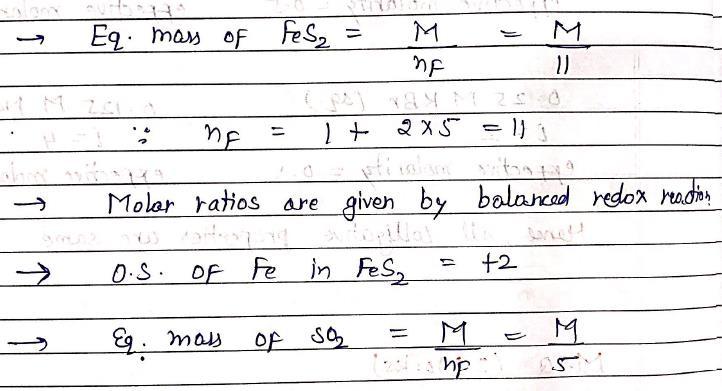*Multiple options can be correct
QUESTION: 33

A drunken person was asked to blow a glass tube packed with acidified potassium dichromate. The change in color of the substance from orange to green confirmed the drinking of alcohol. It is due to:

Solution: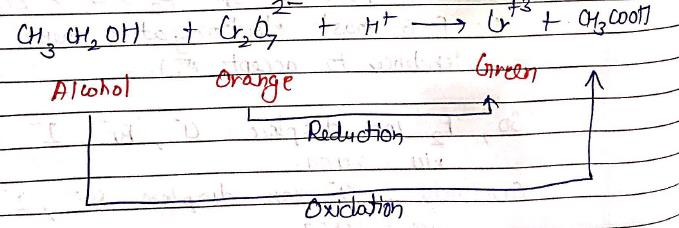*Multiple options can be correct
QUESTION: 34

Which of the following statement(s) is/are correct concerning redox properties ?

Solution: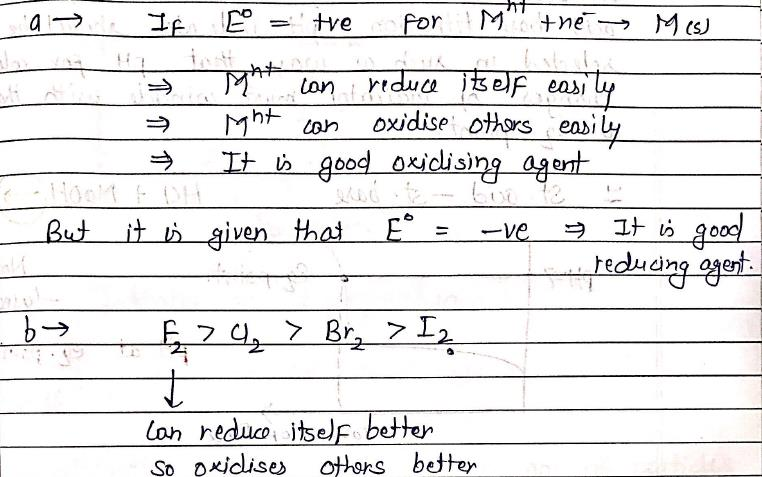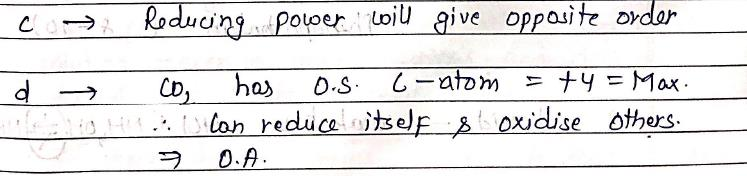*Multiple options can be correct
QUESTION: 35

During titration of HCl and NH4OH the indicator can be used is/are:

Solution: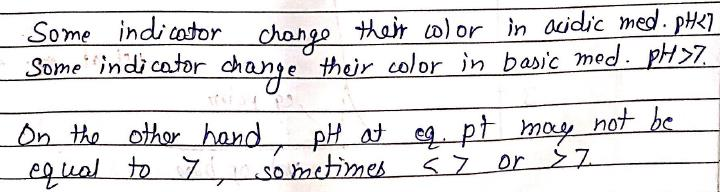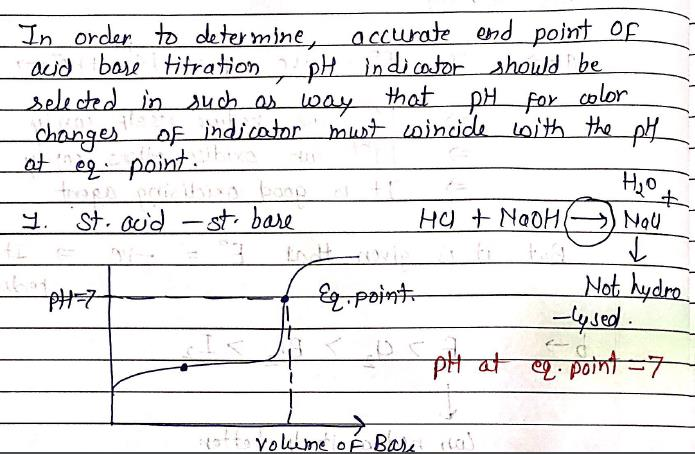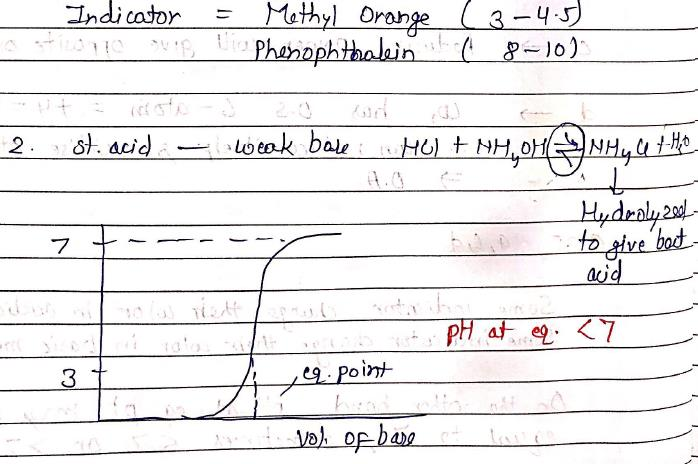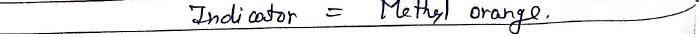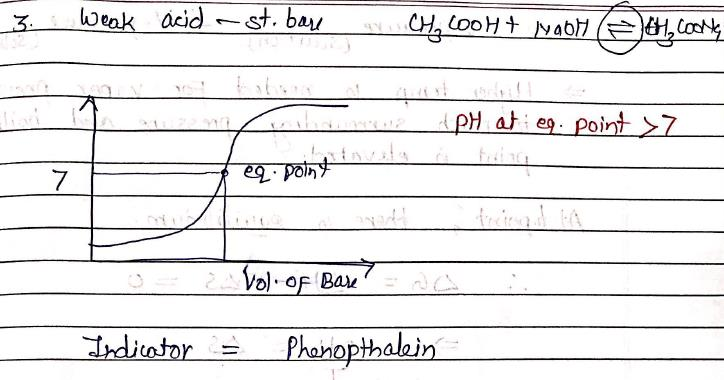*Multiple options can be correct
QUESTION: 36

The higher boiling point of a solution containing non-volatile solute relative to that of a pure solvent is due to:

Solution: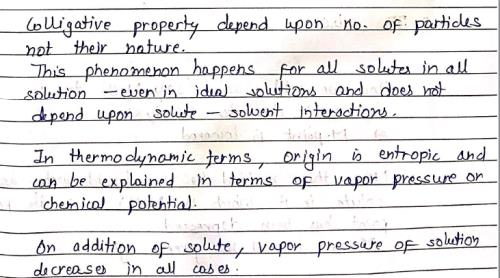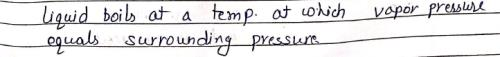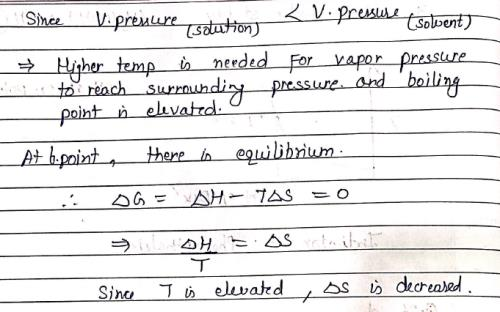*Multiple options can be correct
QUESTION: 37

The use of common salts, e.g. NaCl or CaCl2 anhydrous is made to clear snow on the roads. This causes:

Solution: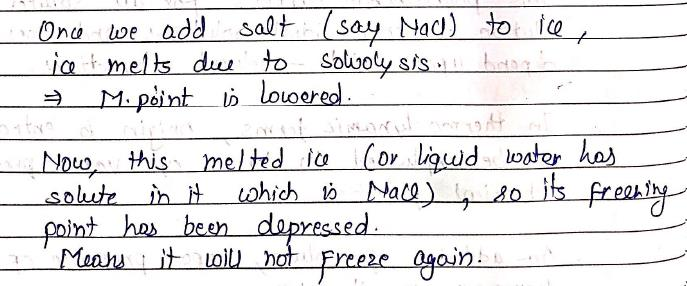*Multiple options can be correct
QUESTION: 38

Consider the following equation: ΔTb = Kbm which of the following is/are incorrect characteristic of Kb?

Solution:*Multiple options can be correct
QUESTION: 39

In the depression of freezing point experiment, it is found that:

Solution:
*Multiple options can be correct
QUESTION: 40

Which of the following statement is/are true ?

Solution:
*Answer can only contain numeric values
QUESTION: 41

What is the oxidation state of Cr in K3CrO8?

Solution: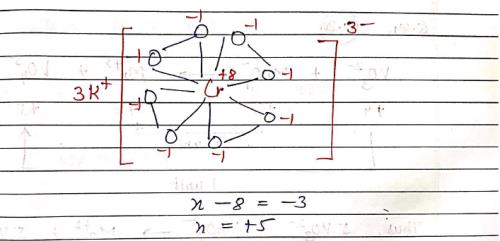*Answer can only contain numeric values
QUESTION: 42

A boy drinks 500 mL of 9% glucose solution. Calculate the number of glucose molecules has consumed (NA = 6.02×1023): [Give answer in the multiple of 1023 rounded up to two decimal places]

Solution: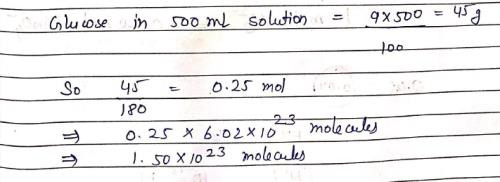*Answer can only contain numeric values
QUESTION: 43

NH3 is oxidized to NO by air (O2) in basic medium. Number of equivalents of NH3 oxidized by 1 mole of O2 is?

Solution: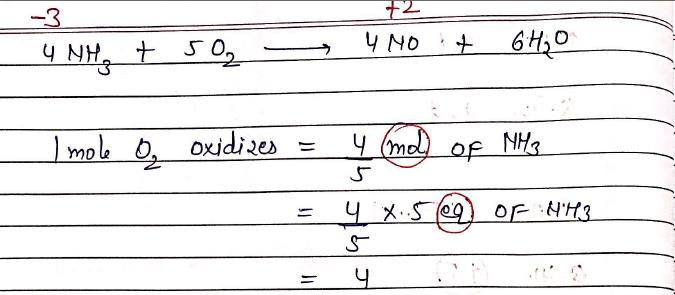*Answer can only contain numeric values
QUESTION: 44

In the reaction, VO32– + MnO4–1 → Mn2+ + VO43–, 1 mole of VO32– is oxidized by how many moles of MnO4? [rounded up to second decimal place]

Solution: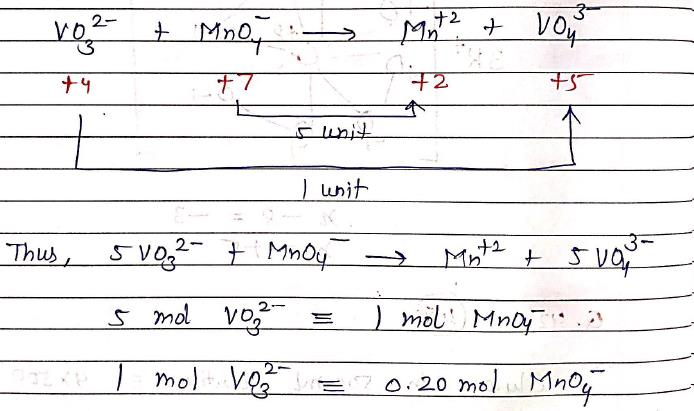*Answer can only contain numeric values
QUESTION: 45

A sodium hydroxide solution has pH = 12. 100 mL of this solution has how many grams of NaOH ? [rounded up to two decimal places]

Solution: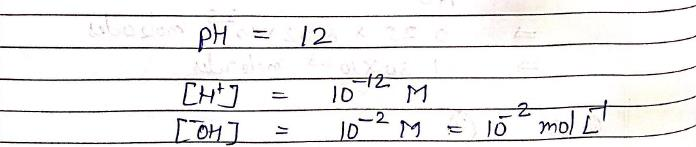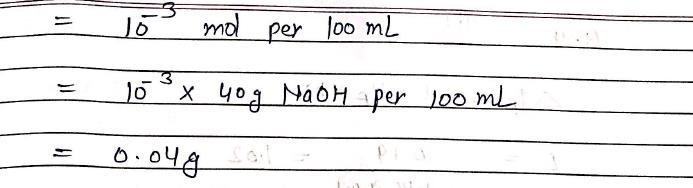*Answer can only contain numeric values
QUESTION: 46

Freezing point of Urea solution is –0.60C. How much Urea (in g) is required to dissolve in 3 Kg of water [Kf = 1.50C Kg/mol]

Solution: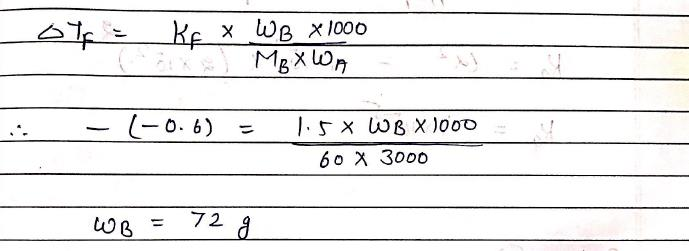*Answer can only contain numeric values
QUESTION: 47

If Na2SO4 is considered to be completely dissociated into ions in aqueous solution, the change in freezing point of water when 0.01 mole of it is dissolved in 1Kg of water, is? [Kf = 1.86 KKg/mol]
[rounded up to three decimal places]

Solution: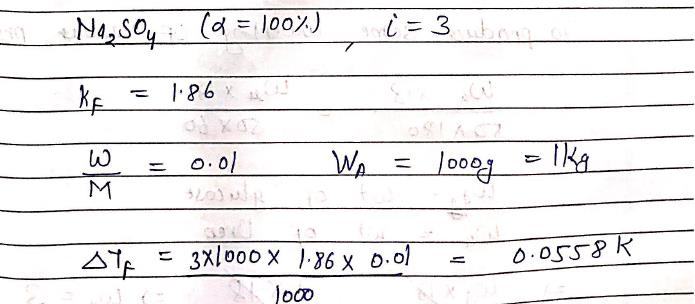*Answer can only contain numeric values
QUESTION: 48

The measured freezing point depression for 0.1m aqueous acetic acid solution is 0.190C. Ka at this concentration will be (Kf = 1.86 K molal–1). [Give answer in multiple of 10–5 rounded up to one decimal places]

Solution: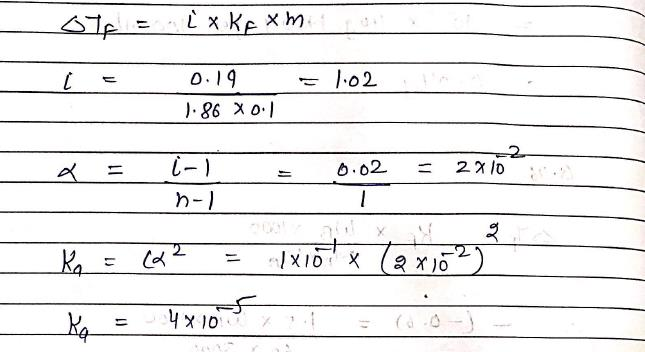*Answer can only contain numeric values
QUESTION: 49

The mass of glucose that should be dissolved in 50 g of water in order to produce same lowering of vapor pressure as is produced by dissolving 1 g of Urea in same quantity of water?

Solution: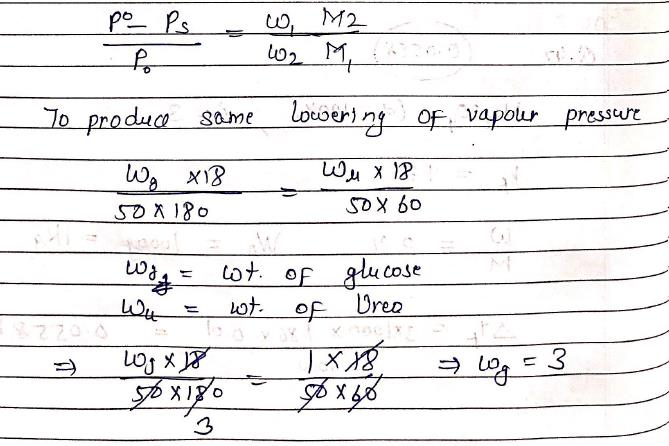*Answer can only contain numeric values
QUESTION: 50

The solution containing 4g of polyvinyl chloride polymer in 1L of dioxane was found to have an osmotic pressure of 6 × 10–4 atm at 300K. Molecular mass of polymer is?
[Give answer in multiple of 105, rounded up to one decimal places]

Solution: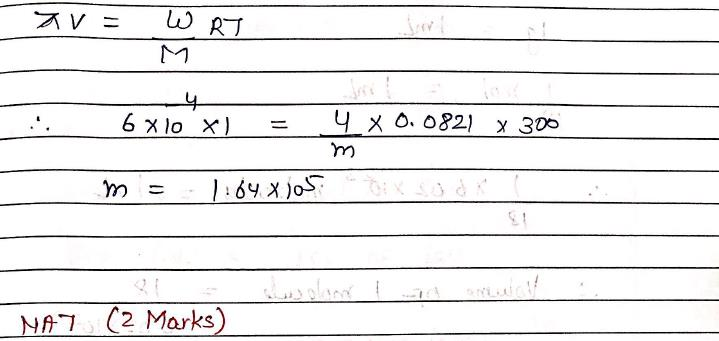*Answer can only contain numeric values
QUESTION: 51

A compound with molecular mass 180 is acylated with CH3COCl to get a compound with molecular mass 390. The number of amino groups present per molecule of the former compound is?

Solution: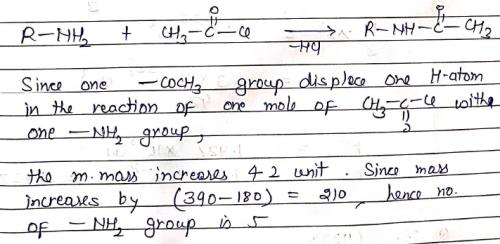*Answer can only contain numeric values
QUESTION: 52

Calculate the radius (Å) of one molecule of water [Assuming it as spherical and having density 1g/mL]. (rounded up to one decimal place)

Solution: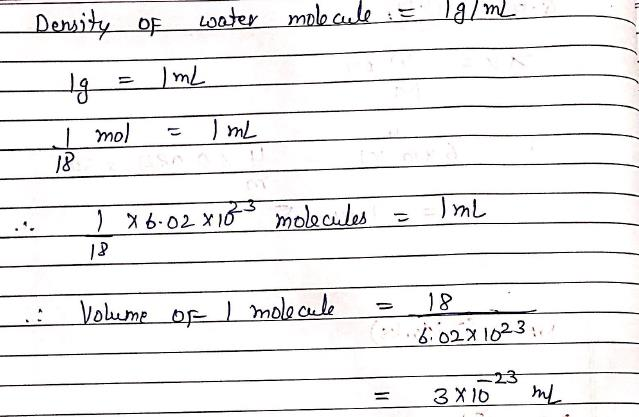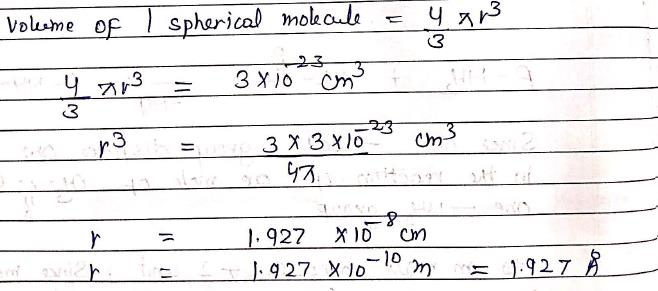*Answer can only contain numeric values
QUESTION: 53

Water gas (CO + H2) consisting of equal volumes of CO and H2 is produced when water vapor is passed over red hot coal. Volume (L) of water gas produced under standard conditions when 3 Kg of coal is treated with water vapor is ? [Vm = 22.4L under standard conditions]

Solution: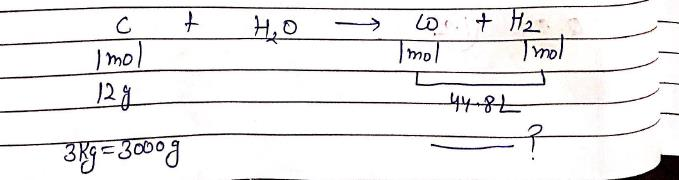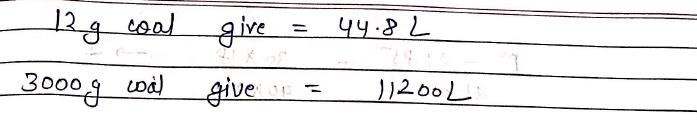*Answer can only contain numeric values
QUESTION: 54

If the reaction of 30 g of CaCO3 produces 15 g of CaO, what is % yield? [rounded up to one decimal place]

Solution: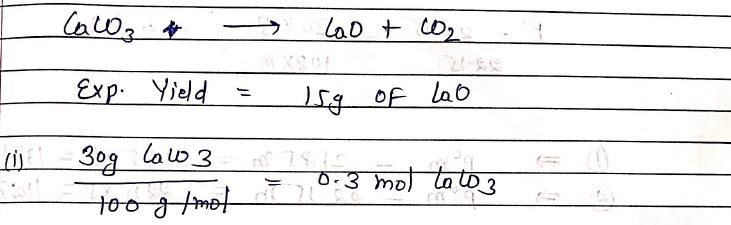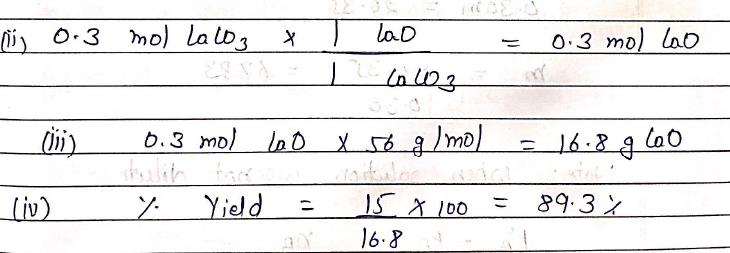*Answer can only contain numeric values
QUESTION: 55

What is oxidation state of P in Ba(H2PO2)2?

Solution: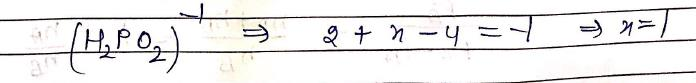*Answer can only contain numeric values
QUESTION: 56

A solution containing 30 g of non-volatile solute in exactly 90 g of water has a vapor pressure of 21.85 mm of Hg at 250 C. Further 18 g of water is added to the solution. The resulting solution has a vapor pressure of 22.15 mm of Hg at same temp. Calculate the molecular weight (g/mol) of solute: [rounded up to two decimal places]

Solution: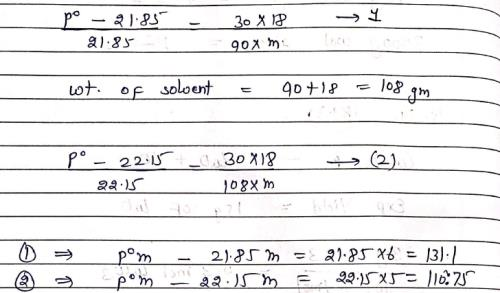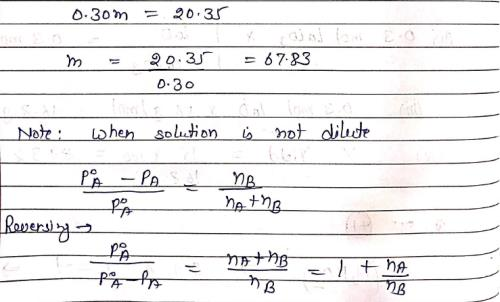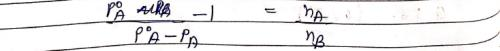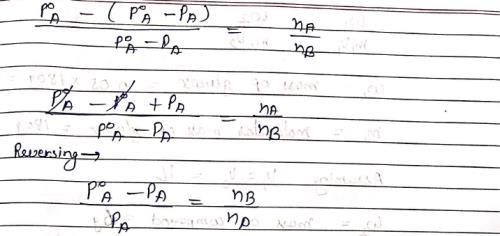*Answer can only contain numeric values
QUESTION: 57

The molar mass of solute sodium hydroxide obtained from measurement of osmotic pressure of its aqueous solution at 270 C is 25 g/mol. Therefore, % ionization is ?

Solution: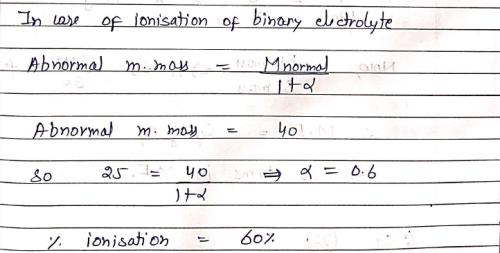*Answer can only contain numeric values
QUESTION: 58

The empirical formula of a non-electrolyte is CH2O. A solution containing 6 g of compound exerts the same osmotic pressure as that of 0.05 M glucose solution at same temp. The molecular formula of compound has …….number of atoms in it:

Solution: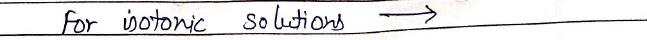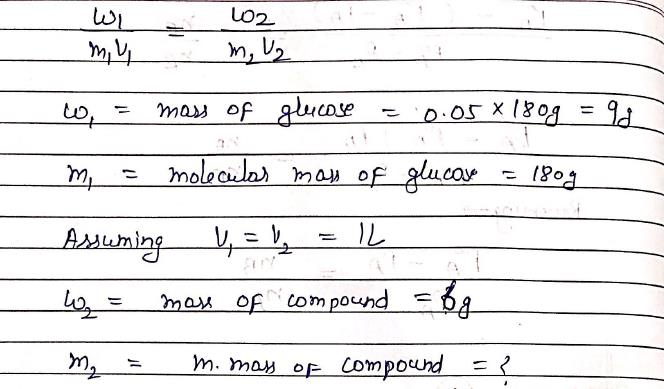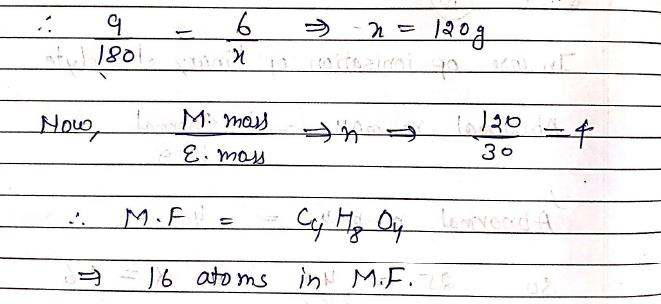*Answer can only contain numeric values
QUESTION: 59

On mixing Heptane and Octane form ideal solution. At 373K, the vapor pressure of two liquid components are 105 and 45 kPa resp. Vapor pressure (kPa) of solution obtained by mixing 25 g of heptane and 35 g of octane will be ? [rounded up to one decimal place]

Solution: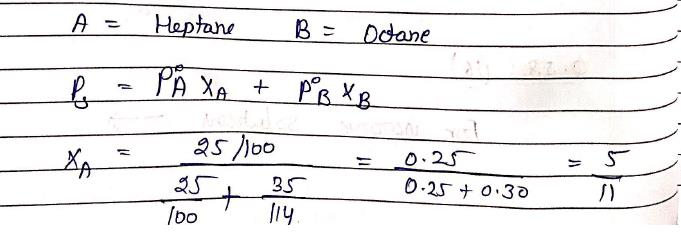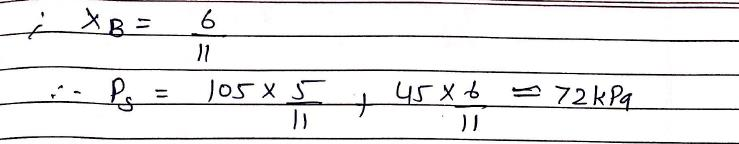*Answer can only contain numeric values
QUESTION: 60

Vapor pressure lowering caused by addition of 100 g of Sucrose (M.Wt. = 342g/mol) to 1000 g of water if vapor pressure of pure water at 250C is 23.8 mmHg: [rounded up to three decimal places]

Solution: